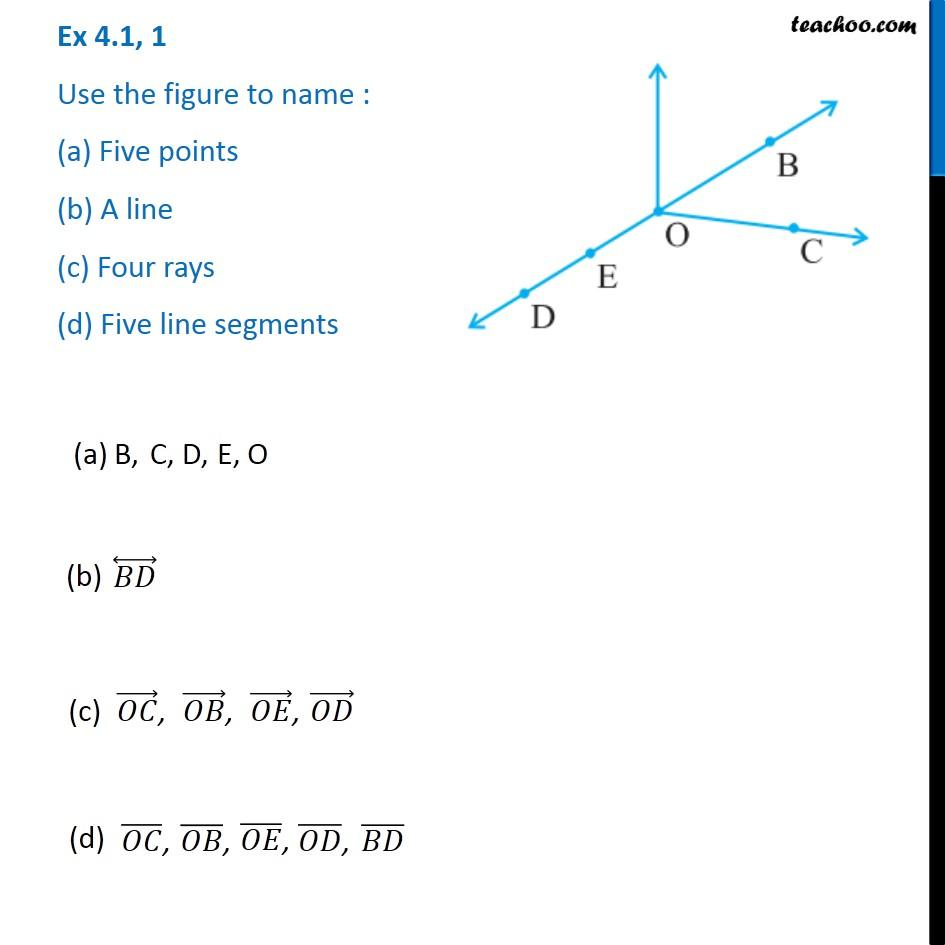1. Chapter 4 Class 6 Basic Geometrical Ideas
2. Concept wise
3. Point, Line, ray, angle

Transcript

Ex 4.1, 1 - Chapter 4 Class 6 - Basic Geometrical Ideas - NCERT Book Use the figure to name : (a) Five points (b) A line (c) Four rays (d) Five line segments The answers are (a) B, C, D, E, O (b) BD (c) OC, OB, OE, OD (d) OC, OB, OE, OD, BD

Point, Line, ray, angle